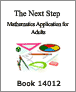Curriculum guidelines

Applications of Best Practices: A Syllabus for Tutors of Independent Studies (I.S.) at Frontier College – Part 1 (2003)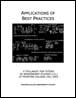Independent Studies (IS) at Frontier College operates adult learning programs, aimed primarily at people with disabilities. This document offers an outline of the main points of the IS course of study.

Authors:
2014-04-29

The Next Step: Mathematics Applications for Adults - Book 14019 (2003)The Book 14019 section of the "Next Step" manual for adults demonstrates the mathematics applications for Number Operations; Fractions, Decimals, and Percent; Percent, Ratio, and Proportion; Geometry; Measurement, Integers; Equations: Equalities and Inequalities; Personal Finance.

2003-08-08

The Next Step: Mathematics Applications for Adults - Book 14018 (2003)The Book 14018 section of the "Next Step" manual for adults demonstrates the mathematics applications for Number Operations; Fractions, Decimals, and Percent; Percent, Ratio, and Proportion; Geometry; Measurement, Integers; Equations: Equalities and Inequalities; Graphs.

2003-08-08

The Next Step: Mathematics Applications for Adults - Book 14017 (2003)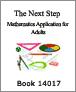The Book 14017 section of the "Next Step" manual for adults demonstrates the mathematics applications for Number Operations; Fractions, Decimals, and Percent; Percent and Ratio; Measurement, Integers; Equations: Equalities and Inequalities; Graphs.

2003-08-08

The Next Step: Mathematics Applications for Adults - Book 14016 (2003)The Book 14016 section of the "Next Step" manual for adults demonstrates mathematics applications for Whole Numbers (number/word recognition, place value, counting, addition, subtraction, multiplication, division, word problems), Fractions (understanding and comparing, addition, subtraction, multiplication and division of fractions, word problems with fractions), Decimals (understanding and comparing, addition, subtraction, multiplication and

2003-08-08

The Next Step: Mathematics Applications for Adults - Book 14015 (2003)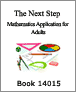The Book 14015 section of the "Next Step" manual for adults demonstrates the mathematics applications for Whole Numbers (Number/Word Recognition, Place Value, Counting, Addition, Subtraction, Multiplication, Division, Word Problems with Whole Numbers, Fractions, Decimals) and Measurement (Time, Money, Charts and Graphs, Metric Measurement, Word Problems with Measurement).

2003-08-08

The Next Step: Mathematics Applications for Adults - Book 14014 (2003)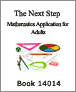The Book 14014 section of the "Next Step" manual for adults demonstrates the mathematics applications for Whole Numbers (Number/Word Recognition, Place Value, Counting, Addition, Subtraction, Multiplication, Division, Word Problems with Whole Numbers, Fractions) and Measurement (Time, Money, Metric Measurement, Word Problems with Measurement).

2003-08-08

The Next Step: Mathematics Applications for Adults - Book 14013 (2003)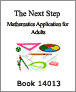The Book 14013 section of the "Next Step" manual for adults demonstrates the mathematics applications for Whole Numbers (Number/Word Recognition, Place Value, Counting, Addition, Subtraction, Multiplication, Division, Word Problems with Whole Numbers, Fractions) and Measurement (Time, Money, Metric Measurement, Word Problems with Measurement).